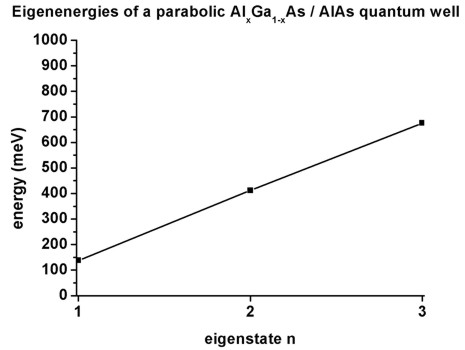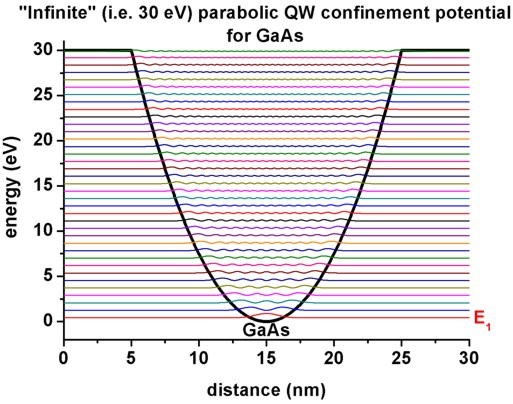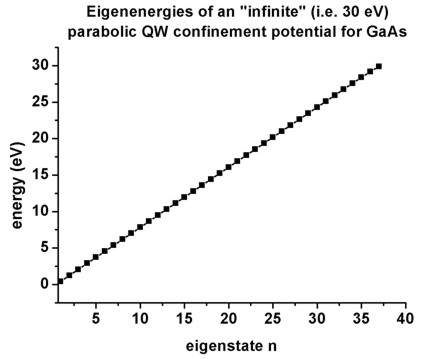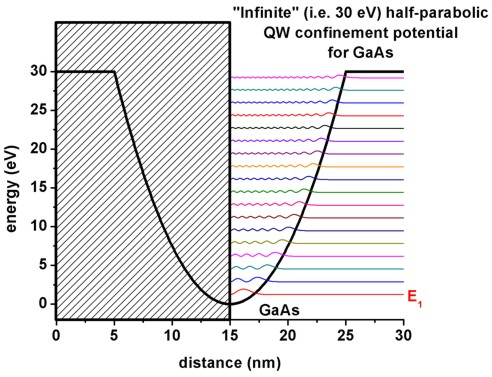nextnano.com  GUI: nextnanomat  Tool: nextnano++  Tool: nextnano³  Tool: nextnano.QCL  Download | Search | Copyright | News | Publications  * password protected nextnano³ software1D Parabolic QW

# nextnano3 - Tutorial

## Parabolic Quantum Well (GaAs / AlAs)

Author: Stefan Birner

If you want to obtain the input files that are used within this tutorial, please contact stefan.birner@nextnano.de.
```-> 1DGaAs_ParabolicQW.in -> 1DGaAs_ParabolicQW_infinite.in -> 1DGaAs_ParabolicQW_infinite_half.in  ```

## Parabolic Quantum Well (GaAs / AlAs)

This tutorial aims to reproduce figures 3.11 and 3.12 (pp. 83-84) of Paul Harrison's excellent book "Quantum Wells, Wires and Dots" (1st edition, Section 3.5 "The parabolic quantum well"), thus the following description is based on the explanations made therein.
We are grateful that the book comes along with a CD so that we were able to look up the relevant material parameters and to check the results for consistency.

### General comments on the solutions of a parabolic potential

An ideal parabolic potential represents a "harmonic oscillator" which is described in nearly every beginner's textbook on quantum mechanics.

The eigenstates can be calculated analytically and are given by the following relationship:

En = ( n - 1/2 )  hbarw0          where n = 1, 2, 3, ...

One feature of a particle that is confined in such a well is that the energy levels are equally spaced by hbarw0 above the zero point energy of 1/2 hbarw0.

The eigenfunctions show an even-odd alternation which is also the case in symmetric, square quantum wells.

The eigenenergies can be measured experimentally by analyzing the optical transitions between the conduction and the valence band states, taking into account the selection rules (both states must have the same parity, see tutorial on interband transitions). For intersubband transitions, different selection rules apply (see tutorial on intersubband transitions). Such an experiment can be used to measure the conduction and valence band offsets because the curvature of the conduction and valence band edges (and thus the eigenstates) depends on the offsets.

(More information on this can be found in The Physics of Low-Dimensional Semiconductors - An Introduction, John H. Davies, Cambridge University Press (1998).)

### Parabolic quantum well:  10 nm AlAs / 10 nm AlGaAs / 10 nm AlAs

`-> 1DGaAs_ParabolicQW.in`

• It is possible to grow parabolic quantum wells by continuously varying the composition of an alloy.
• Our structure consists of a 10 nm AlxGa1-xAs parabolic quantum well (the x alloy content varies parabolically) that is surrounded by 10 nm AlAs barriers on each side.
We thus have the following layer sequence: 10 nm AlAs / 10 nm AlxGa1-xAs / 10 nm AlAs.
The barriers are printed in bold.
• This figure shows the conduction band edge and the three lowest electron wave functions (psi) that are confined inside the parabolic quantum well. All other states are not confined any more.
(Note that the energies were shifted so that the conduction band edge of GaAs equals 0 eV.)The figure is in perfect agreement with Fig. 3.11 (p. 83) of Paul Harrison's book "Quantum Wells, Wires and Dots" (1st edition).

• Technical details:
```  ```The parabolic potential is specified by using a parabolic alloy profile.```  \$material   ...   material-number      = 2   material-name        = Al(x)Ga(1-x)As ! ```AlxGa1-xAs (parabolic quantum well)```   cluster-numbers      = 2   alloy-function       = parabolic  \$end_material  \$alloy-function   ...   material-number      = 2   function-name        = parabolic      ! ```AlxGa1-xAs (parabolic quantum well)```   orientation          = 0 0 1          ! ```along z direction```   vary-from-pos-to-pos = 10d0 20d0      ! ```from 10 nm to 20 nm```   xalloy-from-to       = 0.0d0 1.0d0    ! ```from   Al0.0Ga1.0As = AlAs   to   Al1.0Ga0.0As = GaAs```  \$end_alloy-function  ```

In agreement with Paul Harrison,
- we assumed a constant effective mass of 0.067 m0 throughout the whole sample and
- assumed the conduction band offset between GaAs and AlAs to be 0.83549 eV.

• Output

a) The conduction band edge of the Gamma conduction band can be found here:
`  band_structure / cb1D_001.dat  `The 1st column contains the position in units of` [nm]`.```   ```The 2nd column contains the conduction band edge in units of` [eV]`.``` ```b) This file contains the eigenenergies and the squared wave functions (Psi²):```  Schroedinger_1band / cb001_qc001_sg001_deg001_dir_psi_squared_shift.dat   ```The 1st column contains the position in units of` [nm]`.```   ```(Note that Psin² is shifted with respect to its energy En so that they can be nicely plotted into the conduction band profile.)```   ```This file contains the eigenenergies and the wave functions (Psi):```  Schroedinger_1band / cb001_qc001_sg001_deg001_dir_psi_shift.dat   ```The 1st column contains the position in units of` [nm]`.```   ```(Note that Psin is shifted with respect to its energy En so that they can be nicely plotted into the conduction band profile.)`  `a) and b) can be used to plot the data as shown in the figure above.

c)
This file contains the eigenenergies of the electron states. The units are` [eV]`.```  Schroedinger_1band / ev1D_cb001_qc001_sg001_deg001_dir.dat   ```Paul Harrison uses a 0.01 nm grid whereas we use the 0.01 nm grid only in the middle of the device (or 0.02 nm)
`  `but at the boundaries (i.e. from 0 nm to 5 nm and from 25 nm to 30 nm) we use a 0.1 nm grid to avoid long CPU times:
`  `The eigenvalues read:```      ```nextnano³:   ```       num_ev:  eigenvalue [eV]:                       1        0.1377775566     ```(0.10 / 0.05 / 0.02 / 0.05 / 0.10 nm grid)```                       2        0.4121053675     ```(0.10 / 0.05 / 0.02 / 0.05 / 0.10 nm grid)```                       3        0.6754933822     ```(0.10 / 0.05 / 0.02 / 0.05 / 0.10 nm grid)```                       1        0.1377754485     ```(0.10 / 0.01 / 0.01 / 0.01 / 0.10 nm grid)```                       2        0.4121049460     ```(0.10 / 0.01 / 0.01 / 0.01 / 0.10 nm grid)```                       3        0.6755000401     ```(0.10 / 0.01 / 0.01 / 0.01 / 0.10 nm grid)```      ```Paul Harrison's book: ``` 1        0.1377751623     ```(0.01 nm grid)```                       2        0.4121058503     ```(0.01 nm grid)```                       3        0.6755025905     ```(0.01 nm grid)``` ```Making use of the equation```    ```En = ( n `-` 1/2 )  hbarw0          where n = 1, 2, 3, ... and w0 = (C/m*)1/2
(m* = effective mass, C = constant which is related to the parabolic potential V(z) = 1/2 K z2 )
one can calculate hbarw0:```    ```hbarw0 = 2 E1` ` `- `0 eV = 0.276 eV```    ```hbarw0 =    E2``` ````- `E1    = 0.274 eV```    ```hbarw0 =    E3``` ````- `E2    = 0.263 eV``` ```Obviously, due to the finite AlAs barrier that we have employed, the higher lying states deviate slightly from the analytical results where infinite barriers have been assumed.``` ```This figure shows the eigenenergies for the confined states E1, E2 and E3. As expected they are lying on a straight line because they are separated by hbarw0.The figure is in perfect agreement with Fig. 3.12 (p. 84) of Paul Harrison's book "Quantum Wells, Wires and Dots" (1st edition).

• Matrix elements
The following matrix elements have been calculated:

```intraband-matrix-elements = o !        matrix element < psif* | psii > ```This spatial overlap matrix elements simply returns the Kronecker delta as expected because the wave functions are orthogonal.``` ==> Schroedinger_1band/intraband_o1D_cb001_qc001_sg001_deg001_dir.txt intraband-matrix-elements = p !        matrix element < psif* | p | psii > ==> Schroedinger_1band/intraband_pz1D_cb001_qc001_sg001_deg001_dir.txt ```More details...``` intraband-matrix-elements = z ! dipole matrix element < psif* | z | psii > ==> Schroedinger_1band/intraband_z1D_cb001_qc001_sg001_deg001_dir.txt ```More details...```  ```

## "Infinite" (30 eV) parabolic QW confinement for GaAs

`-> 1DGaAs_ParabolicQW_infinite.in`

• The following figure shows the eigenstates of a parabolic quantum well (GaAs) where the confinement is assumed to be 30 eV.
Now up to 37 eigenstates are confined in the quantum well (grid resolution: 0.025 nm inside the well, 0.05 nm inside the barrier). The figure shows the conduction band profile and the square of the wave functions (psin2) for eigenstate n (n = 1, 2, ..., 37).• This next figure shows the energies of the 37 confined electron states as a funtion of eigenstate n.
As expected, the curve shows a linear dependence because the eigenstates are equally spaced by
hbarw0 = 0.826 eV (where we used` `En = ( n - 1/2 )  hbarw0).```    ```hbarw0 = 2 E1``` ````- `0 eV = 0.8261 eV                E1  / (2 E1)``` = 0.5000    ```hbarw0 =    E2``` ````- `E1    = 0.8260 eV                E2  / (2 E1)``` = 1.4999    ```hbarw0 =    E3``` ````- `E2    = 0.8260 eV                E3  / (2 E1)``` = 2.4997    ```hbarw0 =    E4``` ````- `E3    = 0.8259 eV                E4  / (2 E1)``` = 3.4994    ```hbarw0 =    E5``` ````- `E4    = 0.8259 eV                E5  / (2 E1)``` = 4.4991    ```hbarw0 =    E6``` ````- `E5    = 0.8258 eV                E6  / (2 E1)``` = 5.4987    ```hbarw0 =    E7``` ````- `E6    = 0.8257 eV                E7  / (2 E1)``` = 6.4982    ```hbarw0 =    E8``` ````- `E7    = 0.8257 eV                E8  / (2 E1)``` = 7.4978 ```Still, due to the "infinite" barrier of 30 eV (which is still a finite barrier) that we have employed, the higher lying states deviate slightly from the analytical results where infinite barriers have been assumed. Thus a much higher barrier sho.``` ```• One should bare in mind that the energy level spacing of such parabolic quantum wells is inversely proportional to both the well width and the square root of the effective mass.

• It is also interesting to look at the intraband matrix elements, i.e. to investigate the probability for intersubband transitions.
The relevant output is contained in these two files:
```- Schroedinger_1band / intraband_pz1D_cb001_qc001_sg001_deg001_dir.txt - ```pz``` - Schroedinger_1band / intraband_z1D_cb001_qc001_sg001_deg001_dir.txt  - ```z

From the calculated oscillator strengths it can be seen that only transitions from one level to the neighboring levels (+1 and -1) are allowed.
Because in the case of a harmonic oscillator the momentum operator is proportional to the sum of the creation and the annihilation operators, thus only states can couple that have different occupation numbers with the difference equal to 1.

## "Infinite" (30 eV) half-parabolic QW confinement for GaAs

(Thanks to Michael Povolotskyi who suggested this half-parabolic structure!)

`-> 1DGaAs_ParabolicQW_infinite_half.in`

• The following figure shows the eigenstates when taking only the right half of the parabolic quantum well (GaAs) that has been calculated above. The confinement is 30 eV on the right and infinite confinement on the left (Dirichlet boundary conditions).
Now only 18 eigenstates are confined in the quantum well, i.e. half the number of the eigenvalues compared with the full parabolic QW (grid resolution: 0.025 nm inside the well, 0.05 nm inside the barrier). The figure shows the conduction band profile and the square of the wave functions (psin2) for eigenstate n (n = 1, 2, ..., 18).• Again, the eigenstates are equally spaced. However, the separation energy is now twice the one as before:
hbarw0 = 2 * 0.826 eV = 1.65.``` ```The ground state energy this time is given by:``` ```E1 = ( 3/2 )  hbarw0 / 2.```    ```hbarw0 = 4/3 E1           = 1.639 eV```    ```hbarw0 =    E2``` ````- `E1    = 1.647 eV```    ```hbarw0 =    E3``` ````- `E2    = 1.648 eV```    ```hbarw0 =    E4``` ````- `E3    = 1.648 eV```  ```
• It is also interesting to look at the intraband matrix elements, i.e. to investigate the probability for intersubband transitions.
The relevant output is contained in these two files:
```- Schroedinger_1band / intraband_pz1D_cb001_qc001_sg001_deg001_dir.txt - ```pz``` - Schroedinger_1band / intraband_z1D_cb001_qc001_sg001_deg001_dir.txt  - ```z

• We note that also more realistic parabolic quantum wells can be calculated with nextnano³.
Assuming that the alloy profile is parabolic,
`- `strain can be included (the strain tensor depends on the alloy profile),
`- `as well as effective masses that depend on the alloy profile,
`- `an 8-band k.p model (necessary to get correct intersubband transition energies)
`- `and bowing parameters (especially important for AlGaAs).
All these features are automatically included in the nextnano³ code.

• Please help us to improve our tutorial! Send comments to``` support [at] nextnano.com```.